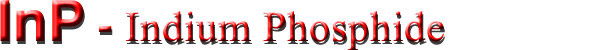## Electrical properties

Basic Parameters
Mobility and Hall Effect
Transport Properties in High Electric Fields
Impact Ionization
Recombination Parameters

### Basic Parameters

 Breakdown field ≈5·105 V cm-1 Mobility electrons ≤5400 cm2V-1s-1 Mobility holes ≤200 cm2 V-1s-1 Diffusion coefficient electrons ≤130 cm2 s-1 Diffusion coefficient holes ≤5 cm2 s-1 Electron thermal velocity 3.9·105 m s-1 Hole thermal velocity 1.7·105 m s-1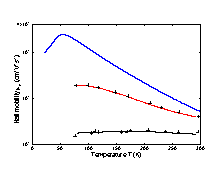Electron Hall mobility versus temperature for different doping levels. Bottom curve - no=Nd-Na=8·1017 cm-3; Middle curve - no=2·1015 cm-3; Top curve - no=3·1013 cm-3. (Razeghi et al. ) and (Walukiewicz et al ).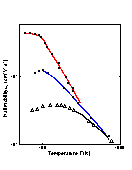Electron Hall mobility versus temperature (high temperatures): Bottom curve - no=Nd-Na~3·1017 cm-3; Middle curve - no~1.5·1016 cm-3; Top curve - no~3·1015 cm-3. (Galavanov and Siukaev).

#### For weakly doped n-InP at temperatures close to 300 K electron drift mobility:

µn = (4.2÷5.4)·103·(300/T) (cm2V-1 s-1)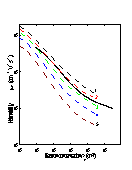Hall mobility versus electron concentration for different compensation ratios. θ = Na/Nd, 77 K. Dashed curves are theoretical calculations: 1. θ = 0; 2. θ = 0.2; 3. θ = 0.4; 4. θ = 0.6; 5. θ = 0.8; (Walukiewicz et al. ). Solid line is mean observed values (Anderson et al. ).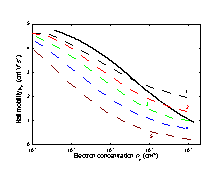Hall mobility versus electron concentration for different compensation ratios θ =Na/Nd, 300 K. Dashed curves are theoretical calculations: 1. θ = 0; 2. θ = 0.2; 3. θ = 0.4; 4. θ = 0.6; 5. θ = 0.8; (Walukiewicz et al. ). Solid line is mean observed values (Anderson et al. ).

#### Approximate formula for electron Hall mobility

µ=µOH/[1+(Nd/107)1/2],
where µOH=5000 cm2V-1 s-1,
Nd- in cm-3 (Hilsum )

At 300 K, the electron Hall factor rn≈1 in n-InP.
for Nd > 1015 cm-3.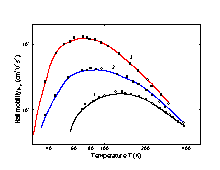Hole Hall mobility versus temperature for different doping (Zn) levels. Hole concentration at 300 K: 1. 1.75·1018 cm-3; 2. 3.6·1017 cm-3; 3. 4.4·1016 cm-3. θ=Na/Nd~0.1. (Kohanyuk et al. ).

#### For weakly doped p-InP at temperature close to 300 K the Hall mobility

µpH~150·(300/T)2.2 (cm2V-1 s-1).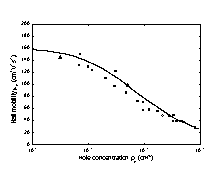Hole Hall mobility versus hole density, 300 K (Wiley ). The approximate formula for hole Hall mobility: µp=µpo/[1 + (Na/2·1017)1/2], where µpo~150 cm2V-1 s-1, Na- in cm-3
At 300 K, the hole factor in pure p-InP: rp~1

### Transport Properties in High Electric Fields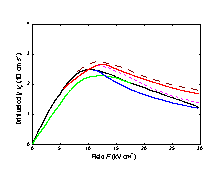Field dependences of the electron drift velocity in InP, 300 K. Solid curve are theoretical calculation. Dashed and dotted curve are measured data. (Maloney and Frey ) and (Gonzalez Sanchez et al. ).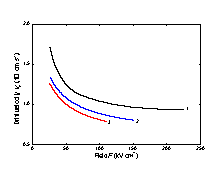The field dependences of the electron drift velocity for high electric fields. T(K): 1. 95; 2. 300; 3. 400. (Windhorn et al. ).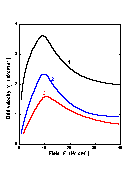Field dependences of the electron drift velocity at different temperatures. Curve 1 -77 K (Gonzalez Sanchez et al. ). Curve 2 - 300 K, Curve 3 - 500 K (Fawcett and Hill ).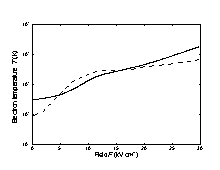Electron temperature versus electric field for 77 K and 300 K. (Maloney and Frey )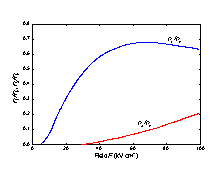Fraction of electrons in L and X valleys nL/no and nX/no as a function of electric field, 300 K. (Borodovskii and Osadchii ).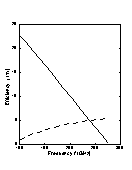Frequency dependence of the efficiency η at first (solid line) and at the second (dashed line) harmonic in LSA mode. Monte Carlo simulation. F = Fo + F1·sin(2π·ft) + F2·[sin(4π·ft)+3π/2], Fo=F1=35 kV cm-1, F2=10.5 kV cm-1 (Borodovskii and Osadchii ).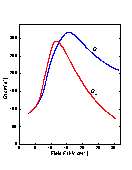Longitudinal (D || F) and transverse (D ⊥ F) electron diffusion coefficients at 300 K. Ensemble Monte Carlo simulation. (Aishima and Fukushima ).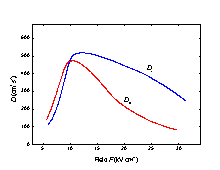Longitudinal (D || F) and transverse (D ⊥ F) electron diffusion coefficients at 77K. Ensemble Monte Carlo simulation. (Aishima and Fukushima ).

### Impact Ionization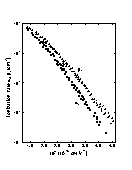The dependence of ionization rates for electrons αi and holes βi versus 1/F, 300 K. (Cook et al. ).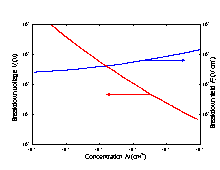Breakdown voltage and breakdown field versus doping density for an abrupt p-n junction, 300 K (Kyuregyan and Yurkov ).

### Recombination Parameters

 Pure n-type material (no ~ 10-14cm-3) The longest lifetime of holes τp ~ 3·10-6 s Diffusion length Lp = (Dp·τp)1/2 Lp ~ 40 µm. Pure p-type material(po ~ 1015cm-3) (a)Low injection level The longest lifetime of electrons τn ~ 2·10-9 s Diffusion length Ln = (Dn·τn)1/2 Ln ~ 8 µm (b) High injection level (filled traps) The longest lifetime of electrons τ ~ 10-8 s Diffusion length Ln Ln ~ 25 µm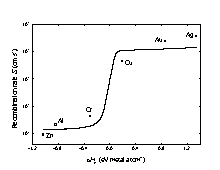Surface recombination velocity versus the heat of reaction per atom of each metal phosphide ΔHR (Rosenwaks et al. ).
If the surface Fermi level EFS is pinned close to midgap (EFS~Eg/2) the surface recombination velocity increases from ~5·10-3cm/s for doping level no~3·1015 cm-3 to ~106 cm/s for doping level no ~ 3·1018cm-3 (Bothra et al. ).
 Radiative recombination coefficient (300 K) 1.2·10-10 cm3/s Auger coefficient (300 K) ~9·10-31 cm6/s